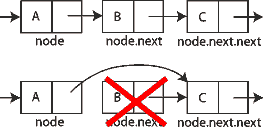# JavaScript 中的链表

## 设计一个链表

``````class LinkedListNode {
constructor(data) {
this.data = data
this.next = null
}
}
``````

``````// create the first node

// add a second node

// add a third node
````````````let current = head

while (current !== null) {
console.log(current.data)
current = current.next
}
``````

## 创建一个单向链表类

``````const head = Symbol('head')

constructor() {
}
}
``````

## 向列表中添加新数据

``````class LinkedList {
constructor() {
}

// create a new node
const newNode = new LinkedListNode(data)

//special case: no items in the list yet
if (this[head] === null) {
// just set the head to the new node
} else {
// start out by looking at the first node
let current = this[head]

// follow `next` links until you reach the end
while (current.next !== null) {
current = current.next
}

// assign the node into the `next` pointer
current.next = newNode
}
}
}
``````

`add()` 方法接受一个参数做为节点的值，并将其添加到列表的末尾。如果列表为空（`this[head]` is null），那么 `this[head]` 等于新添加的节点。如果链表不为空，那么就需要遍历已经存在的链表，找到最后一个节点。遍历使用 while 循环，从`this[head]` 的 next 链接开始，直到找到最后一个节点。最后一个节点的 next 属性为 `null`，停止遍历。然后，您可以将新节点分配给该 next 属性以将数据添加到列表中。

`add()` 方法的复杂度为 O(n)，因为您必须遍历整个列表才能找到插入新节点的位置。除了头部之外，您还可以通过跟踪列表的末尾（通常称为尾部）将这种复杂性降低到 O(1) ，从而允许您立即在正确的位置插入一个新节点。

## 从列表中检索数据

``````class LinkedList {

// other methods hidden for clarity

get(index) {
// ensure `index` is a positive value
if (index > -1) {

// the pointer to use for traversal
let current = this[head]

// used to keep track of where in the list you are
let i = 0

// traverse the list until you reach either the end or the index
while ((current !== null) && (i < index)) {
current = current.next
i++
}

// return the data if `current` isn't null
return current !== null ? current.data : undefined
} else {
return undefined
}
}
}
``````

`get()` 方法首先检查以确保 index 是正值，否则返回 undefined。该 i 变量用于跟踪遍历进入列表的深度。循环本身与您之前看到的基本遍历相同，但添加了循环应在 i 等于 index 时退出 。这意味着有两种情况可以退出循环：

1. current is null，这意味着 index 大于列表长度。
2. i 等于 index，表示 current 是该 index 位置的节点。

`get()` 方法的复杂度从移除第一个节点时的 O(1)（不需要遍历）到移除最后一个节点时的 O(n)（需要遍历整个列表）。复杂度很难再降低，因为始终需要进行搜索以识别要返回的正确值。

## 从链表中删除数据remove 操作其实就是两个操作：

1. 查找指定的索引（与 `get()` 中的算法相同）
2. 删除该索引处的节点

• 列表为空（无法遍历）
• 索引小于零
• 索引大于列表中的项目数
• 索引为零（去掉头部）

``````class LinkedList {

// other methods hidden for clarity

remove(index) {
// special cases: empty list or invalid `index`
if ((this[head] === null) || (index < 0)) {
throw new RangeError(`Index \${index} does not exist in the list.`)
}

// special case: removing the first node
if (index === 0) {
// temporary store the data from the node
const data = this[head].data

// just replace the head with the next node in the list

// return the data at the previous head of the list
return data
}

// pointer use to traverse the list
let current = this[head]

// keeps track of the node before current in the loop
let previous = null

// used to track how deep into the list you are
let i = 0

// same loops as in `get()`
while ((current !== null) && (i < index)) {

// save the value of current
previous = current

// traverse to the next node
current = current.next

// increment the count
i++
}

// if node was found, remove it
if (current !== null) {

// skip over the node to remove
previous.next = current.next

// return the value that was just removed from the list
return current.data
}

// if node wasn't found, throw an error
throw new RangeError(`Index \${index} does not exist in the list.`)
}
}
``````

`remove()` 方法首先检查两个特殊情况，一个空列表 (this[head] is null) 和一个 index 小于零的列表。在这两种情况下都会抛出错误。

`remove()` 方法的复杂度与 `get()` 移除第一个节点时的 O(1) 到移除最后一个节点时的 O(n)相同。

## 使列表可迭代

`values()` 方法只需要对列表和 yield 每个节点包含的数据做一个基本的遍历：

``````class LinkedList {

// other methods hidden for clarity

* values() {
let current = this[head]

while (current !== null) {
yield current.data
current = current.next
}
}

[Symbol.iterator]() {
return this.values()
}
}
``````

`values()` 方法用星号 (*) 标记以表明它是一个生成器方法。该方法遍历列表，yield 用于返回它遇到的每条数据。（请注意，该 Symbol.iterator 方法未标记为生成器，因为它从 `values()` 生成器方法返回一个迭代器。）

## 使用链表

``````const list = new LinkedList()

// get the second item in the list
console.log(list.get(1)) // "orange"

// print out all items
for (const color of list) {
console.log(color)
}

// remove the second item in the list
console.log(list.remove(1)) // "orange"

// get the new first item in the list
console.log(list.get(1)) // "yellow"

// convert to an array
const array1 = [...list.values()]
const array2 = [...list]
``````

## Keywords

`JavaScript` `链表`